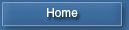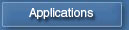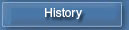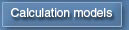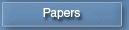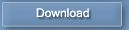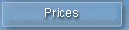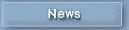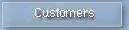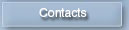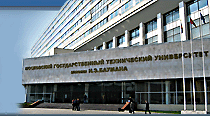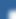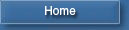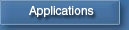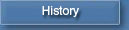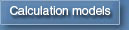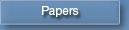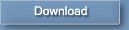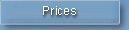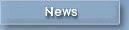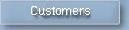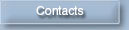Diesel-RK in Telegram## Simulation of Soot formation in diesel

Method for simulation of soot formation and burning in diesel cylinder was developed by Dr., Prof. Razleitsev /1/. Method takes into account features of sprayed fuel burning. It is assumed, the soot is formed mainly by two ways:

• As a result of chain destructive transformation of molecules of fuel diffusing from the surface of drops to the front of a flame.
• Owing to high-temperature thermal polymerization and dehydrogenization of a vapor-liquid core of evaporating drops.

In parallel to this, the process of burning of soot particles and reduction of their volumetric concentration owing to expansion occurs.

Detailed description of equations is presented in /1/, therefore only end formulas are presented in this page.

Soot formation rate in a burning zone is: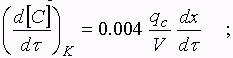where: V is a current volume of cylinder;
qc is a cycle fuel mass;
dx/dt is a heat release rate.

Soot formation rate due to high-temperature thermal polymerization of drops nucleuses is proportionate to rate of disappearance of drops because of full evaporation. In different processes one is calculated with different equations.

In injection period: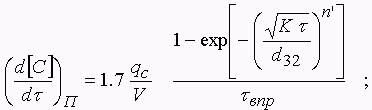where: t  is a current time from injection beginning,
t âďđ is an injection duration,
n'  is a factor of distribution (for diesels injectors:  n' = 2..4),
K is a constant of evaporation,
d32 is a mean Sauted diameter of drops.

After injection termination: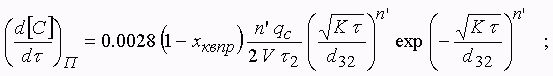where: t 2 is a current time after injection termination;
xkâďđ is a fraction of heat released at the end of injection.

Rate of soot burning: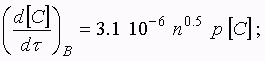where: p is a current cylinder pressure, MPa,
[C] = C / V is a current soot concentration in cylinder.

Rate of soot concentration decrease because of expansion: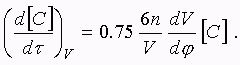Resulting rate of soot formation and burning: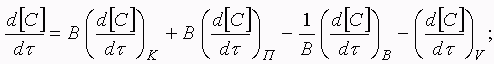where: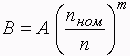is an empiric factor,
n  is RPM,
níîě is RPM at full capacity,
A, m are calibrate coefficients.

Exhaust gas soot concentration related to normal conditions: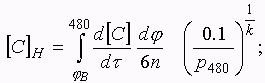where: p480 is a cylinder pressure at 60o before BDC,
k is an exhaust gas adiabatic exponent (1.33).

Hartridge smoke level is: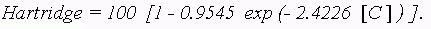Alike equations are used to calculate Bosch smoke number and Factor of Absolute Light Absorption: K, m-1.

Particulate Matter emission is calculated by equation of Alkidas /2/ as a function of Bosch smoke number: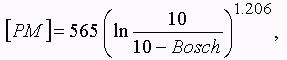Results of simulation of soot release rate in cylinder of truck diesel KamAZ 7405 are presented in fig. 1.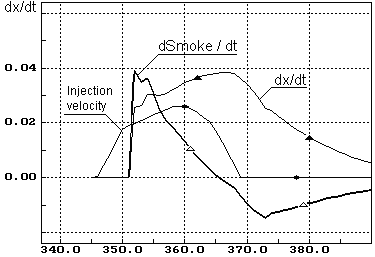Fig. 1. Soot release rate in cylinder of truck diesel KamAZ 7405
(RPM=2200;  BMEP=9.5 bars)

A comparison of calculated engine parameters with measured ones at operating of engine in external torque curve is presented in fig. 2. Injection profiles were specified for each operating point of performance.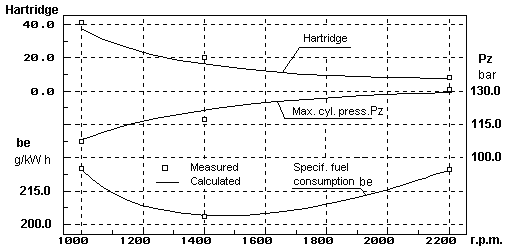Fig. 2. External performance of truck diesel KamAZ 7405
Measured data were presented by KamAZ   R&D Center.

Bibliography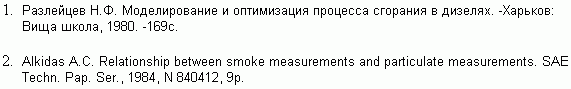To page "Calculation models"